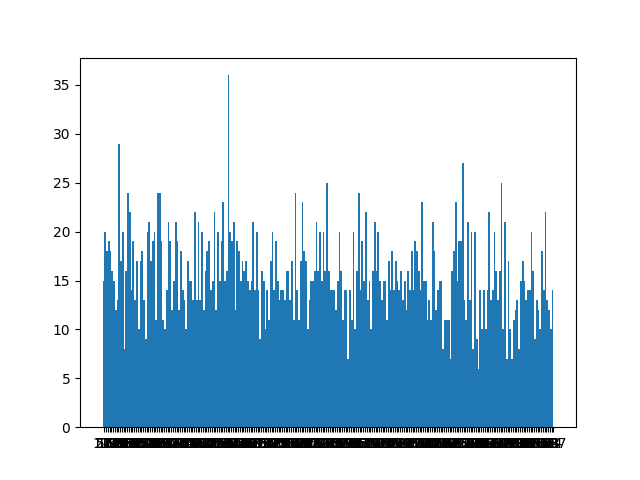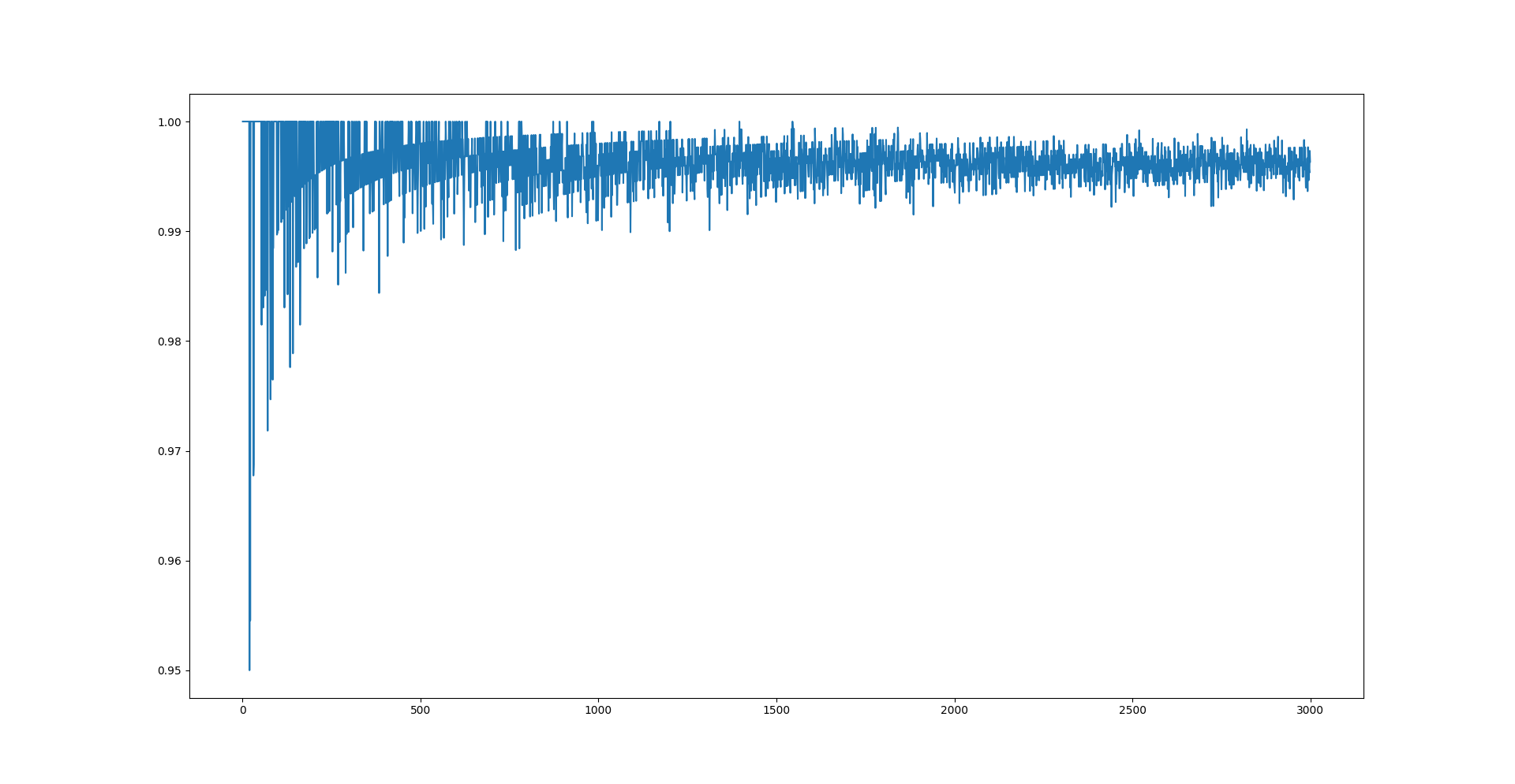# Permutations

TJCTF 2018 - Cryptography (70 pts).

# TJCTF 2018: Permutations

### Challenge details

Event Challenge Category Points Solves
TJCTF 2018 Permutations Cryptography 70 17

### TL;DR

A biais in RC4 PRNG that make us able to recover one byte of the plaintext message with statiscal analysis coupled to permutation of the message make us able to recover the initial message.

### Methology

We first got a python file without knowing which cryptograpy was in it ( no clues in the chall presentation about it ). After a quick look at it we find that the cipher algorithm is RC4 (Wikipeda)

#!/usr/bin/env python3
from os import urandom
from itertools import permutations

def ksa(key):
permS = []
for x in range(256):
permS.append(x)
j = 0
for x in range(256):
j = (j+permS[x]+key[x%16])%256
temp = permS[x]
permS[x] = permS[j]
permS[j] = temp
return permS
def prga(S, mes):
x = 0
y = 0
mes = bytearray(mes, "utf_8")
cipher = ""
stream = bytearray()
for z in range(len(mes)):
x = (x+1)%256
y = (y+S[x])%256
temp = S[x]
S[x] = S[y]
S[y] = temp
stream.append(S[(S[x]+S[y])%256])
op = str(hex(mes[z]^stream[z]))
if len(op)%2 != 0:
cipher += "0"+op[2:]
else:
cipher += op[2:]
return cipher
message = xxxxxxxxxx
out=""
return out
while True:
print("Enter a permutation of abcdefghij and I'll encrypt the corresponding message! ")
i = input()
em = change(i)
key = urandom(16)
tS = ksa(key)
ct = prga(tS, em)
print(ct)


#### First Step : Finding the flaw

The first thing I did, was to compare the implemented algorithm with the real algorithm of the RC4 and they wre the same.

Then I looked at the change function :

def change(maskmes):
out=""
return out


This function simply does a permutation according to the order of the alphabet :

• If maskmes value is “acbdefghij”, the second letter of the message will be interverted with the third
• If maskmes value is “jabcdefghi”, the last letter of the message will be the first

So for the moment there is no flaws in this function. After searching for almost 3 or 4 hours we found ( thks @ENOENT ) the line of text that made the attack possible.

The best such attack is due to Itsik Mantin and Adi Shamir who showed that the second output byte of the cipher was biased toward zero with probability 1128 (instead of 1256). This is due to the fact that if the third byte of the original state is zero, and the second byte is not equal to 2, then the second output byte is always zero. Such bias can be detected by observing only 256 bytes.

Wikipedia

So the second byte of the RC4 Pseudo Random Generation Algorithm is biaised an tend more often to zero than the other results.

#### Second step : Exploiting It

Now that we have a clue about what to do, let’s find how to use it.

op = str(hex(mes[z]^stream[z]))


In the implementation the PRNG is used as a keystream to Xor the message with it. The fact is that when you take any letter k, k^0 = k. So ig the zero byte appear more often for the second byte, the second character of the plaintext will appear more often than the others in the ciphertext.

I did some test to determine how much time we need to cipher it to find with good frequency the plain character.

Here is the occurences of each characters at the second position for 4000 ciphers generated :Here is the frequency of apparition according to the number of ciphertext.We can clearly see on the first diagram that there is a more occurent character. And the second show us that we will need approximatively 4000 iterations to have a good percentage of finding the clear character.

#### Final Step : Let’s script it !

I used Pwntools to make my socket connection easier to implement.

#!/usr/bin/env python2.7
from pwn import *
from collections import Counter

HOST = "problem1.tjctf.org"
PORT = 8007

initial_permutation = "abcdefghij"
current_permutation = ""
flag = ""
for j in range(10):
r = remote(HOST,PORT)
result = ""
current_permutation = list(initial_permutation)
current_permutation =  current_permutation[j]
current_permutation[j] = "b"
for i in range(4000):
r.recv()
r.sendline(''.join(current_permutation))
letter = r.recvline().strip().decode("hex")
result += letter
print("%d--%s"%(i,letter))
char, occur = Counter(result).most_common(1)
log.success("Current flag : %s"%(flag+char))
flag += char
r.close()
log.success(flag)


tjctf{ohbyteRC4!}

@Areizen